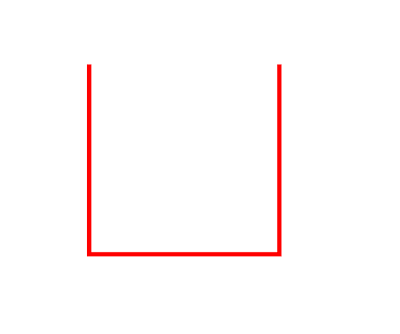Sunday, February 2, 2014

Constructing a Space-filling Curve

This is the second part of a three-part post. For the first, see here.

The previous post introduced the concept of a curve in the plane, presenting it as a type of mapping from one-dimensional to two-dimensional space, with the added condition that it be continuous. Finally, an interesting mapping that fills the unit square was presented. However, this example is not continuous. To show this, we shall demonstrate that a particular sequence {xn} = {x1,x2,x3,...} of points in t-space (the one-dimensional domain of the mapping) converges to a point x, also in the domain of f. Then, we shall show that the corresponding sequence of points {f(x1),f(x2),f(x3),...} does not converge to the image f(x) of the limit of the sequence. This will prove that the mapping is discontinuous.

Let {xn} be the sequence {0.490000...,0.499000...,0.499900...,...}. Clearly this sequence converges to x = 0.500. The sequence in the unit square {(0.400...,0.900...),(0.490...,0.900...),(0.490...,0.990...)}, whose members correspond to the value of f at each member of {xn} converges to (0.500...,0.999...), but the value of f at x is not this value. Rather it is f(0.500...) = (0.500...,0.000...). Thus f is not a continuous mapping. Note that even if the non-terminating decimal expansions were selected for those members of the unit interval where there are two possibilities, the function would still be discontinuous, and one would this time use a sequence of points approaching t = 1/2 from above rather than below.

Though, as mentioned previously, Peano was the first to demonstrate the existence of a continuous space-filling curve, the process described below actually refers to a space-filling curve devised by Hilbert shortly afterward, as it is more straightforward geometrically.

To actually construct a space-filling curve f that is continuous, we make use of a limiting process, which goes through steps called iterations. The first iteration of the process that results in the curve is as shown below:This is the image of the first iteration of the mapping in the plane, but the way it is constructed is more easily understood when the square is divided into four smaller squares. The part of the curve lying in each smaller square is the image of one-quarter of the unit interval, as illustrated below.Each subset of the unit interval marked with a Roman numeral is mapped to the corresponding red segment contained within the square marked by the same numeral. Clearly the parametrization of the red curve defined this way is continuous, and is a mapping of the unit interval into the unit square. Also, at each point, the segment is equidistant from the center and edge of the square, so each of the three straight parts is of length 1/2.

The next step is to apply the limiting process. The second iteration of the curve is essentially several smaller connected copies of the first, as shown below.The second iteration of the limiting process (the blue curve at bottom) consists of four copies of the first iteration (some have been rotated) and segments connecting them so that the curve is continuous. Instead of mapping 1/4 of the unit interval into the segment contained within each square we now cut the interval into 16 regions and map each to the part of the curve within each of the 16 squares of side length 1/4 in the proper order. The first eight squares are numbered by Roman numerals in the diagram.

The process then continues, with each step consisting of four copies of the previous one, rotated in the same manner. On the nth step of the process, we can visualize the mapping as taking each subset of the unit interval of length 1/4n to the portion of the curve contained within a square of side length 1/2n, of which there are 4n in total.

Note in addition that the curve of the first iteration is composed of three segments of length 1/2, and thus has total length 3/2. Since the second iteration is made up of copies of the first scaled down by a factor of 1/2 (to fit in the squares which have half the sidelength of the original), it is made of 12 segments of length 1/4, plus 3 additional segments of this length to connect the copies. Thus the total length of the curve of the second iteration is 15/4. Generally, it is easy to see that, taking into account the segments that must be attached to the copies of previous iterations to connect them, that the length of the nth iteration is 2n - 1/2n. This sequence of lengths increases without bound, and therefore the end result, the Hilbert curve, is a curve of infinite length contained within a finite area.

In the next post, we prove that the Hilbert curve indeed fills the unit square and explore more of its properties and extensions.

Counterexamples in Analysis by Bernard R. Gelbaum and John M. H. Olmsted, Hilbert Curve on Wikipedia